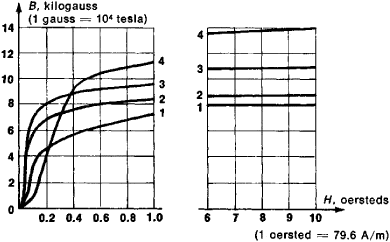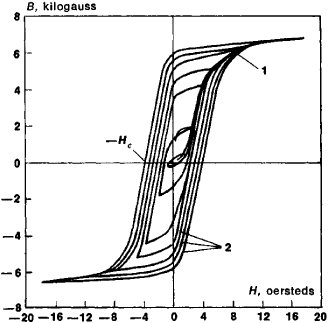# Magnetization Curve

## magnetization curve

[‚mag·nəd·ə′zā·shən ‚kərv]
(electromagnetism)
McGraw-Hill Dictionary of Scientific & Technical Terms, 6E, Copyright © 2003 by The McGraw-Hill Companies, Inc.
The following article is from The Great Soviet Encyclopedia (1979). It might be outdated or ideologically biased.

## Magnetization Curve

a graph, table, or formula that shows the dependence of the magnetization J or magnetic induction B on the magnetic-field intensity H. If the function J(H) is known, then the induction curve B(H) can be constructed for the same substance, since the simultaneous values of B, J, and H that refer to the same volume element of the substance are related by the equation B = H + 4∏J (in the centimeter-gram-second system of units) or B = μ0 (H + J) (in SI units, where μ0 is the magnetic constant).

The magnetization curves for magnetic materials depend not only on the physical properties of the materials and the external conditions but also on the sequence of passage through various magnetic states. In this connection, we consider several types of magnetization curves: (1) curves of initial magnetization (FigureFigure 1. Curves of initial magnetization of permalloys: (1) chromium permalloy, (2) molybdenum permalloy, (3) permalloy with 75.8 percent Ni and the remainder Fe, (4) permalloy with 45 percent Ni and the remainder Fe

1), which are the sequences of values of J or B that a substance assumes as H increases monotonically from an initial state with B = H = J = 0 (in this case, H does not change direction); (2) cyclic magnetic-reversal curves (or static hysteresis loops), which are the functional forms of B(H) or J(H) that are obtained after repeated passage through a certain range of values of H in the forward and reverse directions (Figure 2); and (3) normal magnetization (or commutation) curves, which are the loci of the tips of the symmetric loops of magnetic reversal (Figure 2).Figure 2. Family of symmetric hysteresis loops (2) and the normal magnetization curve (1) for molybdenum permalloy (79 percent Ni, 4 percent Mo, 0.2 percent Mn, the remainder Fe)

Magnetization curves enable us to determine the characteristics of magnetic materials, for example, residual magnetization, coercive force Hc, and magnetic permeability. These characteristics are used in design calculations for the magnetic circuits of electromagnets, magnetic starters, relays, and other electrotechnical devices and instruments.

### REFERENCES

Kifer, 1.1. Ispytaniia ferromagnitnykh materialov, 3rd ed. Moscow, 1969.
Bozorth, R. Ferromagnetizm. Moscow, 1956. (Translated from English.)
The Great Soviet Encyclopedia, 3rd Edition (1970-1979). © 2010 The Gale Group, Inc. All rights reserved.
References in periodicals archive ?
We observed that the [Fe.sub.3][O.sub.4] powder with particle size between 50 and 60 nm exhibits a strong magnetization curve as shown in Figure 4(a).
According to a technical paper, (4) "Harmonics are created through the hysteresis of the magnetization curve of a part inside a test coil.
Caption: Figure 4: Normalized magnetization curve at the value of 1T of the ([gamma]-[Fe.sub.2][O.sub.3])-Mn nanoparticles as a function of Mn concentration recorded at 300 K.
At C = 2 at.%, the magnetization curve achieved saturation by the maximum of saturation of [M.sub.s] = 1.1 emu/g.
Caption: FIGURE 5: Schematic diagram of B-H magnetization curve.
The corresponding magnetization curve of porous [Fe.sub.3][O.sub.4] powder as a function of magnetic field, or the M vs H, is shown in Fig.
This design method ensures that the operating zone coincides with the linear region of the magnetization curve avoiding saturation, thus presenting a contribution to the development of multilevel power converters.
A material is called magnetically anisotropic if the magnetization curve of the material depends on the direction to which the material is magnetized.

Site: Follow: Share:
Open / Close